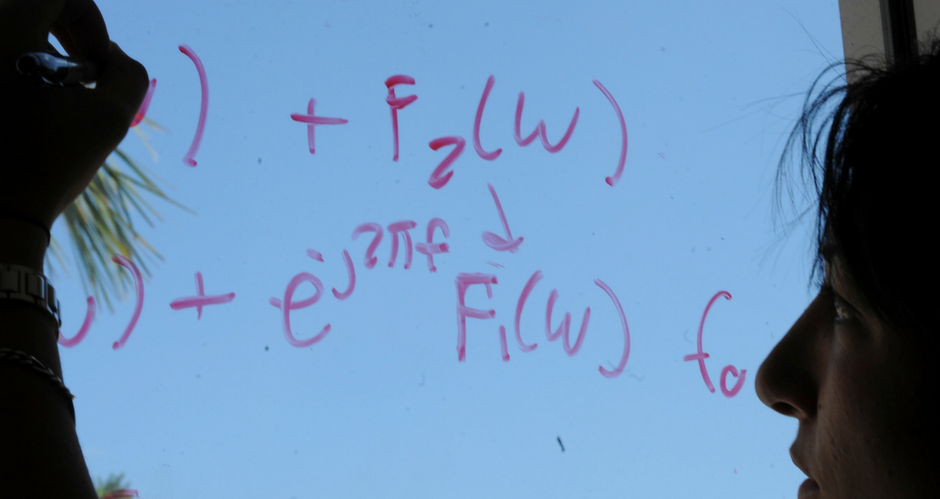## Mathematics and System Engineering Student Publications

Poster

#### Abstract

onsider the non-homogeneous heat equation with Neumann and Robin boundary conditions: (See Poster for the equations (1)-(4)). The problem (1)-(4) is well posed for sufficiently well behaved data, but what if some of the data is not known, for example, (See Poster for formulas) are unknown. This inverse problem can be formulated as an optimal control problem, as follows.

2015